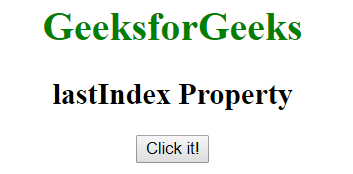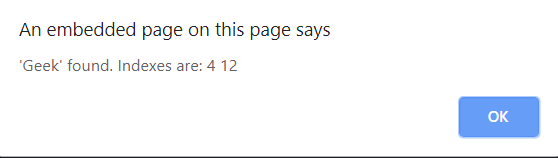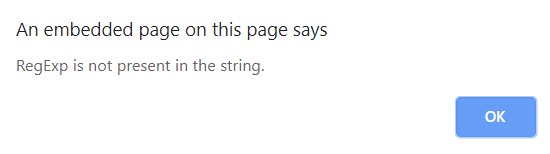# JavaScript | lastIndex Property

The lastIndex Property in JavaScript is used to specify the index at which to start the next match. If “g” modifier is not present this property will not work.
It can be used to return the position of character immediately after the previous match.

Syntax:

`RegexObj.lastIndex`

Example-1: This example checks if the string contains “Geek” in it.

 ` ` `<``html``> ` ` `  `<``head``> ` `    ``<``title``> ` `      ``lastIndex Property ` `  `` ` ` ` ` `  `<``body` `style``=``"text-align:center"``> ` `    ``<``h1` `style``=``"color:green"``> ` `      ``GeeksforGeeks ` `  `` ` `    ``<``h2``> ` `      ``lastIndex Property ` `  `` ` `    ``<``button` `onclick``=``"geek()"``> ` `      ``Click it! ` `  `` ` ` `  `    ``<``script``> ` `        ``function geek() { ` `            ``var str =  ` `                ``"GeeksforGeeks is the"+ ` `                ``" computer science portal"+ ` `                ``" for geeks"; ` `           `  `            ``var patt1 = /Geek/g; ` `            ``var ans = "'Geek' found. Indexes are: "; ` `            ``// check "geek" in string. ` `            ``while (patt1.test(str) == true) { ` `                ``ans += patt1.lastIndex + " "; ` `            ``} ` `            ``alert(ans); ` `        ``} ` `    `` ` ` ` ` `  ` `

Output:
Before clicking the button:After clicking the button:Example-2: This example checks if the string contains “abc” in it.

 ` ` `<``html``> ` ` `  `<``head``> ` `    ``<``title``> ` `      ``lastIndex Property ` `  `` ` ` ` ` `  `<``body` `style``=``"text-align:center"``> ` `    ``<``h1` `style``=``"color:green"``> ` `      ``GeeksforGeeks ` `  `` ` `    ``<``h2``>lastIndex Property ` `    ``<``button` `onclick``=``"geek()"``> ` `      ``Click it! ` `  `` ` ` `  `    ``<``script``> ` `        ``function geek() { ` `            ``var str =  ` `                ``"GeeksforGeeks is"+ ` `                ``" the computer science"+ ` `                ``" portal for geeks"; ` `           `  `            ``var patt1 = /abc/g; ` `            ``var ans =  ` `                ``"'Geek' found. Indexes are: "; ` `            ``var ans1 =  ` `                ``"'Geek' found. Indexes are: "; ` `            ``var len = ans.length; ` `           `  `            ``// check "geek" in string. ` `            ``while (patt1.test(str) == true) { ` `                ``ans1 += patt1.lastIndex + " "; ` `            ``} ` `            ``var x = ans1.length; ` `            ``if (len === x) ` `                ``alert("RegExp is not "+ ` `                      ``"present in the string."); ` `            ``else ` `                ``alert(ans1); ` `        ``} ` `    `` ` ` ` ` `  ` `

Output:
Before clicking the button:After clicking the button:Supported Browsers: The browsers supported by JavaScript lastIndex Property are listed below: